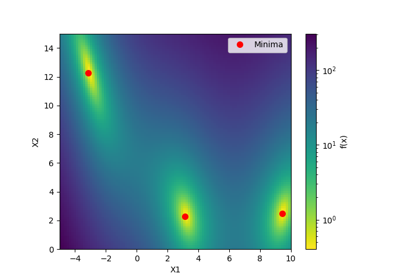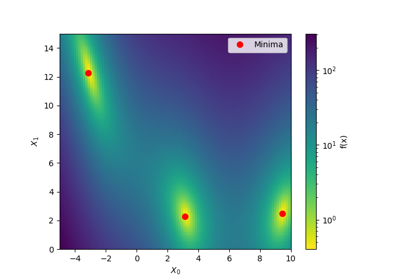# skopt.dummy_minimize¶

skopt.dummy_minimize(func, dimensions, n_calls=100, initial_point_generator='random', x0=None, y0=None, random_state=None, verbose=False, callback=None, model_queue_size=None, init_point_gen_kwargs=None)[source][source]

Random search by uniform sampling within the given bounds.

Parameters
funccallable

Function to minimize. Should take a single list of parameters and return the objective value.

If you have a search-space where all dimensions have names, then you can use skopt.utils.use_named_args() as a decorator on your objective function, in order to call it directly with the named arguments. See use_named_args for an example.

dimensionslist, shape (n_dims,)

List of search space dimensions. Each search dimension can be defined either as

• a (lower_bound, upper_bound) tuple (for Real or Integer dimensions),

• a (lower_bound, upper_bound, prior) tuple (for Real dimensions),

• as a list of categories (for Categorical dimensions), or

• an instance of a Dimension object (Real, Integer or Categorical).

n_callsint, default: 100

Number of calls to func to find the minimum.

initial_point_generatorstr, InitialPointGenerator instance, default: "random"

Sets a initial points generator. Can be either

• "random" for uniform random numbers,

• "sobol" for a Sobol sequence,

• "halton" for a Halton sequence,

• "hammersly" for a Hammersly sequence,

• "lhs" for a latin hypercube sequence,

• "grid" for a uniform grid sequence

x0list, list of lists or None

Initial input points.

• If it is a list of lists, use it as a list of input points.

• If it is a list, use it as a single initial input point.

• If it is None, no initial input points are used.

y0list, scalar or None

Evaluation of initial input points.

• If it is a list, then it corresponds to evaluations of the function at each element of x0 : the i-th element of y0 corresponds to the function evaluated at the i-th element of x0.

• If it is a scalar, then it corresponds to the evaluation of the function at x0.

• If it is None and x0 is provided, then the function is evaluated at each element of x0.

random_stateint, RandomState instance, or None (default)

Set random state to something other than None for reproducible results.

verboseboolean, default: False

Control the verbosity. It is advised to set the verbosity to True for long optimization runs.

callbackcallable, list of callables, optional

If callable then callback(res) is called after each call to func. If list of callables, then each callable in the list is called.

model_queue_sizeint or None, default: None

Keeps list of models only as long as the argument given. In the case of None, the list has no capped length.

Returns
resOptimizeResult, scipy object

The optimization result returned as a OptimizeResult object. Important attributes are:

• x [list]: location of the minimum.

• fun [float]: function value at the minimum.

• x_iters [list of lists]: location of function evaluation for each iteration.

• func_vals [array]: function value for each iteration.

• space [Space]: the optimisation space.

• specs [dict]: the call specifications.

• rng [RandomState instance]: State of the random state at the end of minimization.

For more details related to the OptimizeResult object, refer http://docs.scipy.org/doc/scipy/reference/generated/scipy.optimize.OptimizeResult.html

## Examples using skopt.dummy_minimize¶